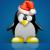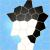php连接符和输出问题

jht727415 发布于 2015/08/29 17:05

年薪达不到25W大数据工程师、拿不到Offer全额退款->>>echo '5+1=' . 1+5;  //输出10

1.和+的运算符优先级相同，LZ的语句结合顺序就是从左到右。

'5+1=' . 1会先执行，导致最终结果就是 '5+1=1'+5，+号前面强制转换成5了。

“.”换成”,“，“,”优先级最低，会先“+”。

0//由于
echo (int)'5+1='; // 5
echo '5+1' . 1;   // 5+11
echo (int)('5+1=' . 1);   // 5
echo (int)('5+1=' . 1) + 5;  // 10
// 所以
echo '5+1=' . 1+5; // 10

0

echo '5+1=' . 1+5;  // 输出10，错误

echo '5+1=' . 5+1;
// 输出6，正确